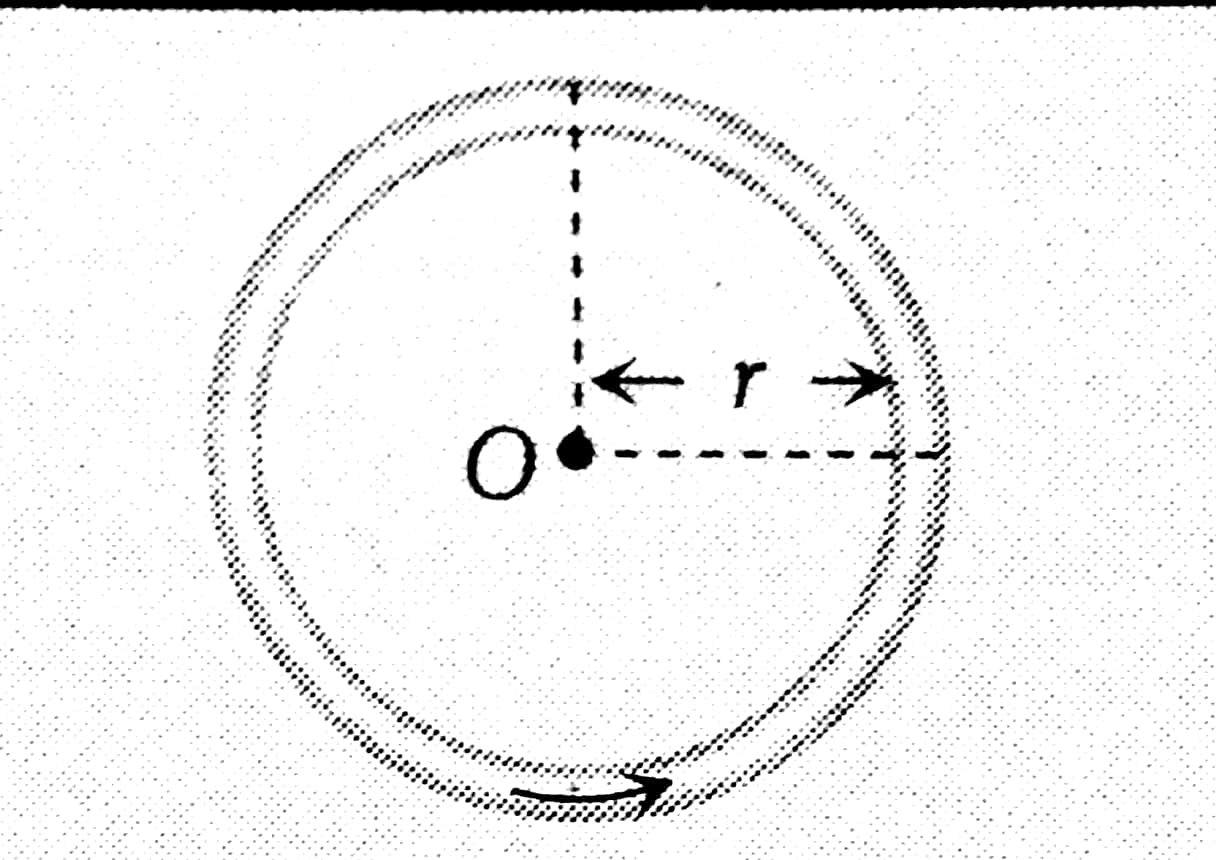Q

# Solve! At the centre of a fixed large circular coil of radius R, a much smaller circular coil of radius r is placed. The two coils are concentric and are in the same plane. The larger coil carries a current I. The smaller coil is set to rotate with a

At the centre of a fixed large circular coil of radius R, a much smaller circular coil of radius r is placed. The two coils are concentric and are in the same plane. The larger coil carries a current I. The smaller coil is set to rotate with a constant angular velocity ω about an axis along their common diameter. Calculate the emf induced in the smaller coil after a time t of its start of rotation.

• Option 1)

• Option 2)

• Option 3)

• Option 4)

812 Views

As we learnt

Magnetic field due to cCrcular Current Carrying arc -

- whereinMagnetic field due to cCrcular Current Carrying arc -

- whereinAt time the samller coil is rotated by

Angle between

Option 1)

Option 2)

Option 3)

Option 4)

Exams
Articles
Questions